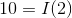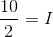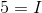# SAT II Physics : Ohm's Law

## Example Questions

### Example Question #1 : Electricity

If a circuit contains a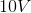battery and a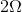resistor what is the current of the circuit?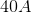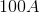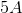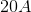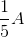Explanation:

Ohm's law states: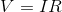Whereis voltage,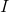is current, andis resistance.

We can substitute the given values from the question into the equation and solve: